# Vector and Vector Resolution Scalar Vector Vectors Vector

• Slides: 29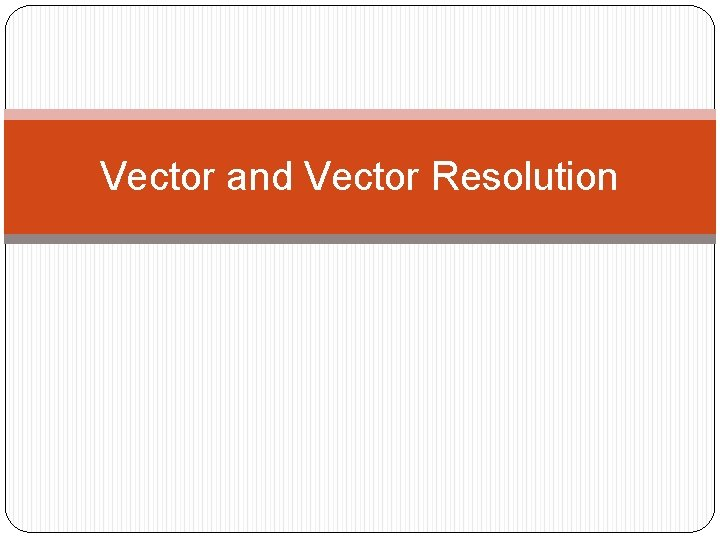Vector and Vector ResolutionScalarVectorVectors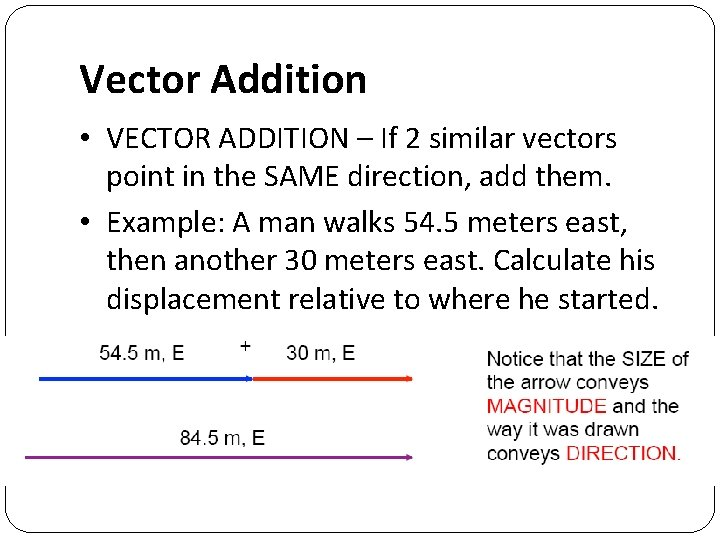Vector Addition • VECTOR ADDITION – If 2 similar vectors point in the SAME direction, add them. • Example: A man walks 54. 5 meters east, then another 30 meters east. Calculate his displacement relative to where he started.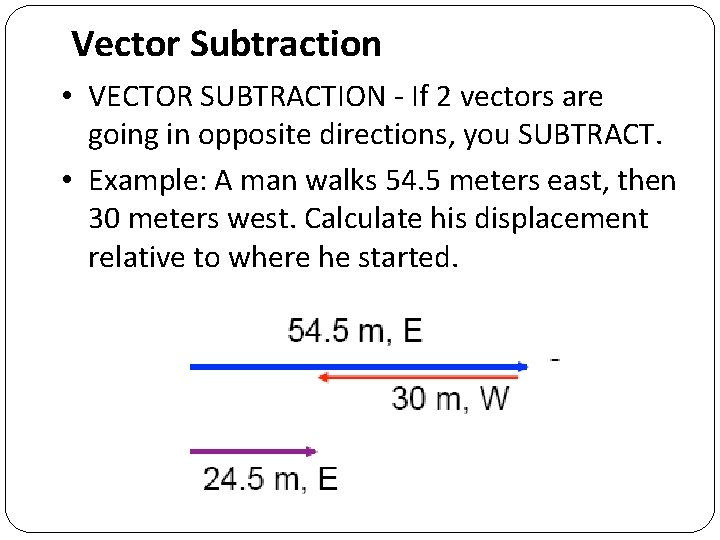Vector Subtraction • VECTOR SUBTRACTION - If 2 vectors are going in opposite directions, you SUBTRACT. • Example: A man walks 54. 5 meters east, then 30 meters west. Calculate his displacement relative to where he started.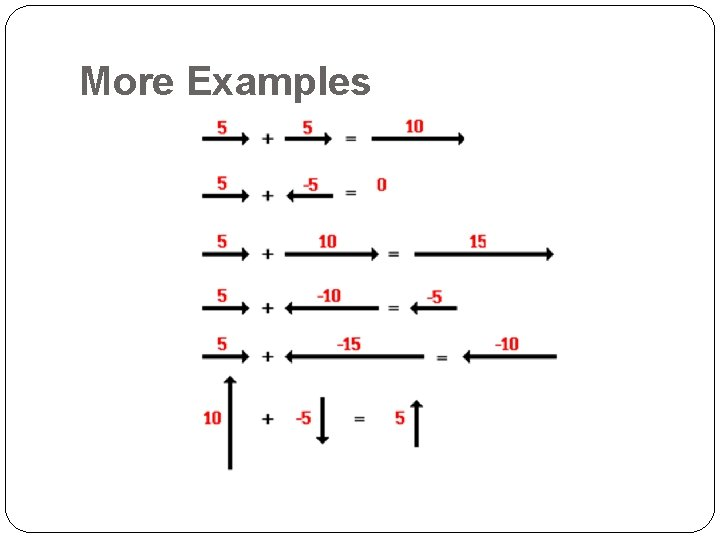More ExamplesVectors Are Typically Drawn to Scale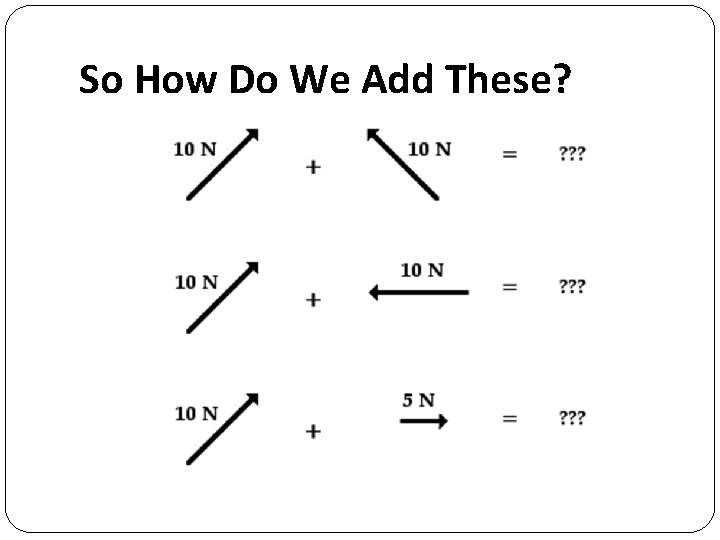So How Do We Add These?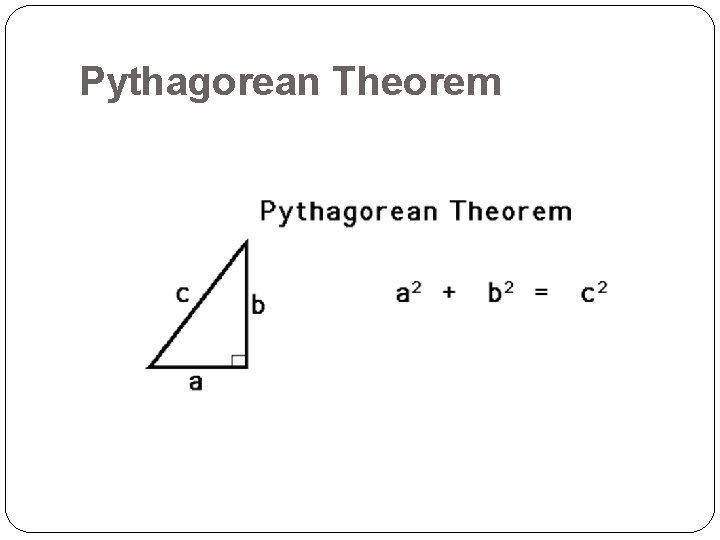Pythagorean Theorem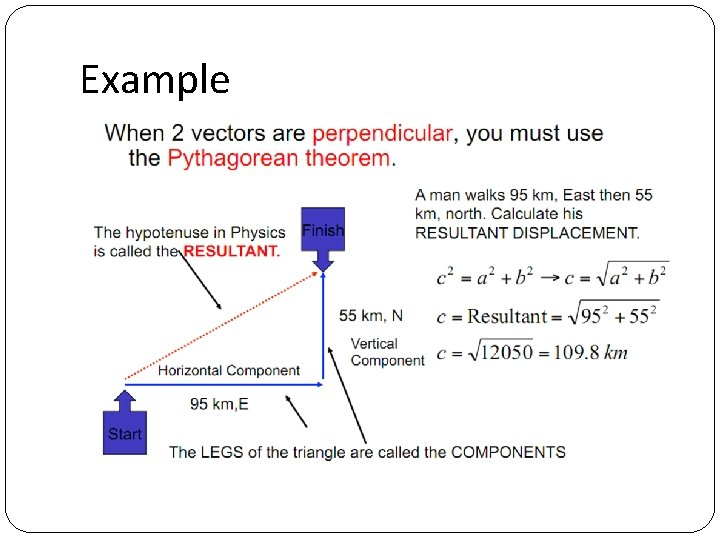Example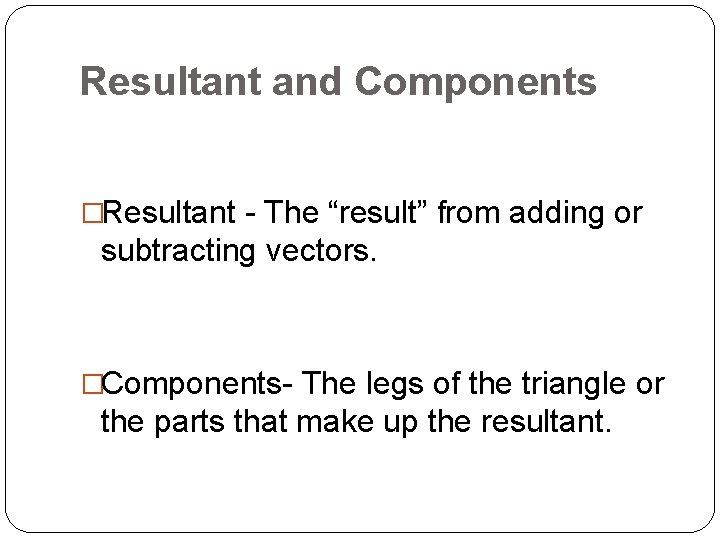Resultant and Components �Resultant - The “result” from adding or subtracting vectors. �Components- The legs of the triangle or the parts that make up the resultant.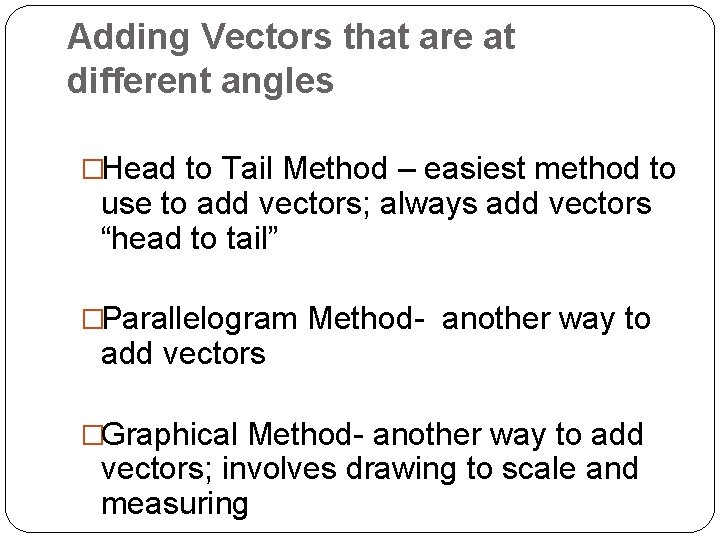Adding Vectors that are at different angles �Head to Tail Method – easiest method to use to add vectors; always add vectors “head to tail” �Parallelogram Method- another way to add vectors �Graphical Method- another way to add vectors; involves drawing to scale and measuring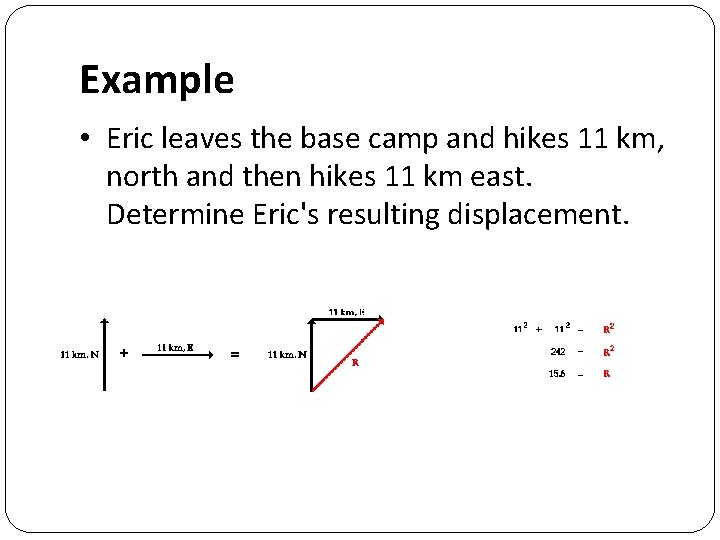Example • Eric leaves the base camp and hikes 11 km, north and then hikes 11 km east. Determine Eric's resulting displacement.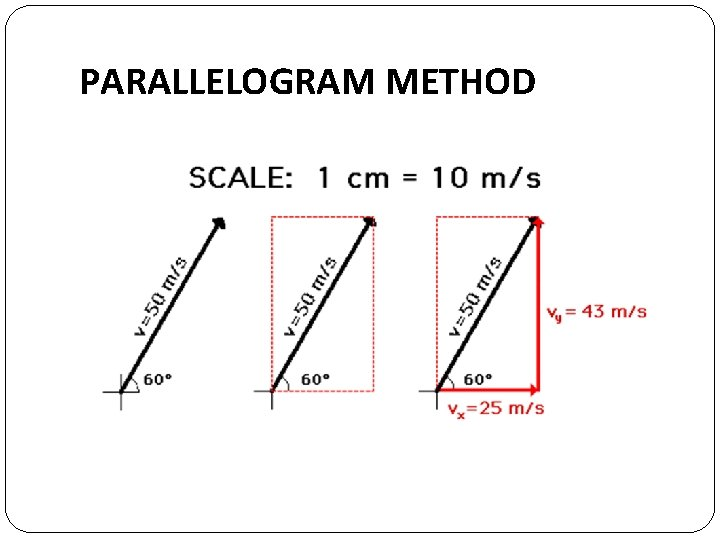PARALLELOGRAM METHOD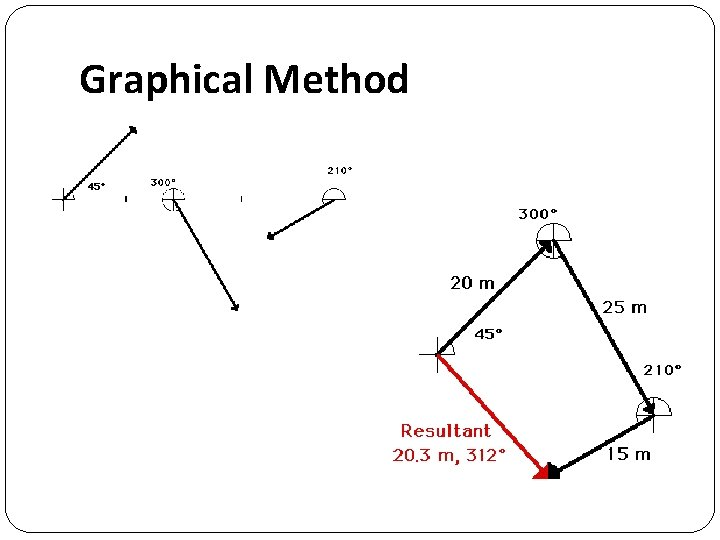Graphical Method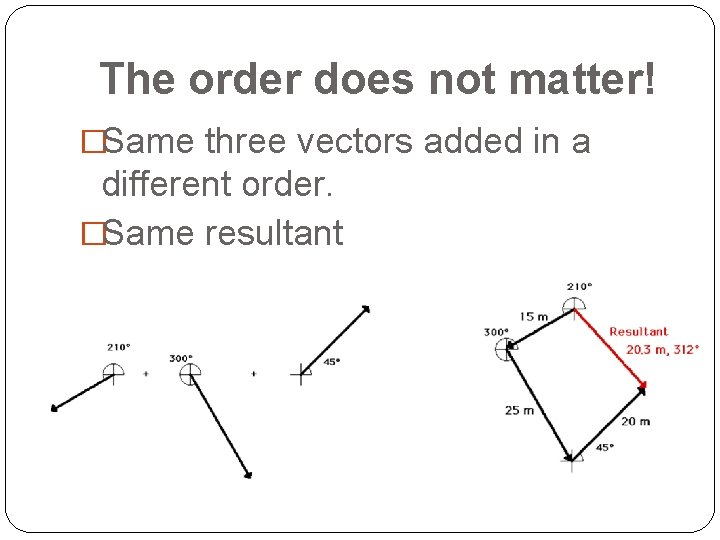The order does not matter! �Same three vectors added in a different order. �Same resultant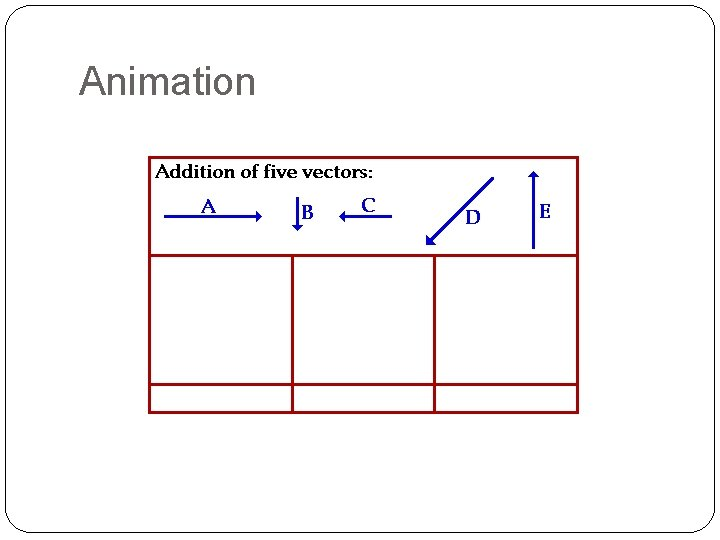Animation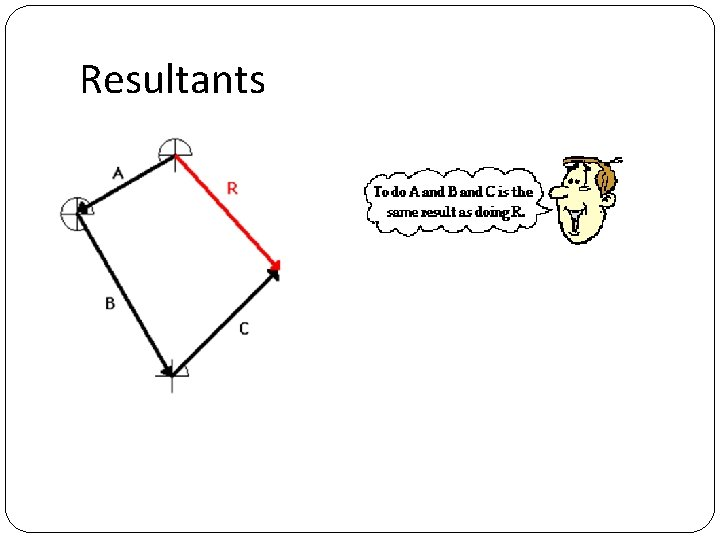ResultantsTail Wind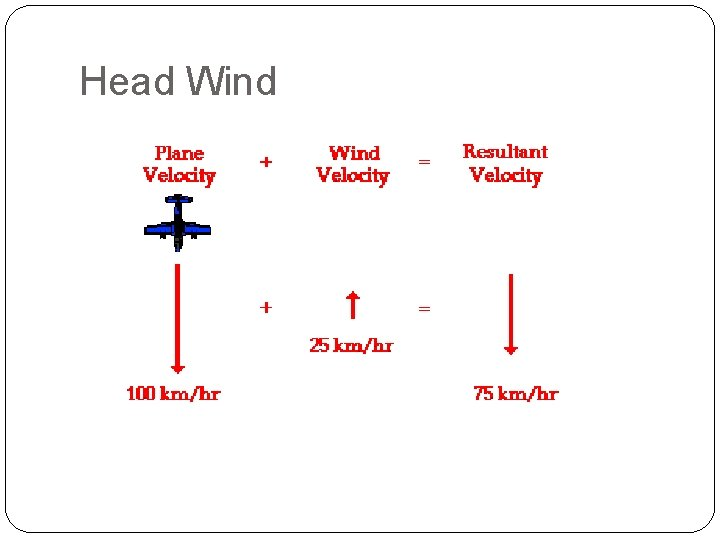Cross Wind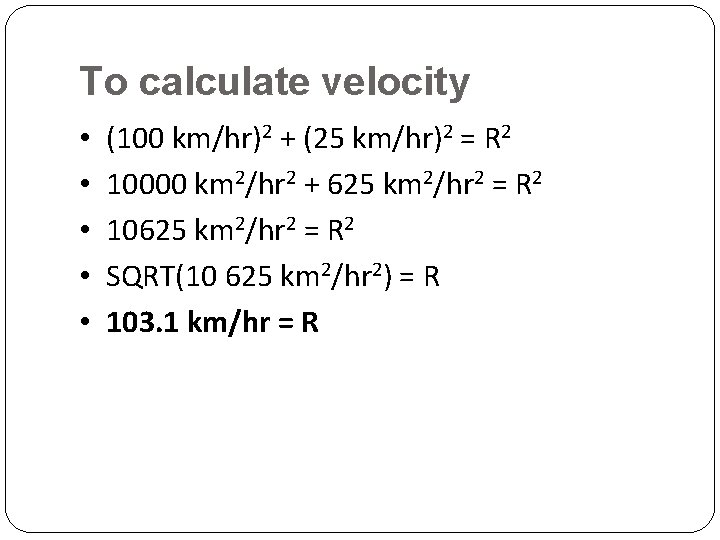To calculate velocity • • • (100 km/hr)2 + (25 km/hr)2 = R 2 10000 km 2/hr 2 + 625 km 2/hr 2 = R 2 10625 km 2/hr 2 = R 2 SQRT(10 625 km 2/hr 2) = R 103. 1 km/hr = R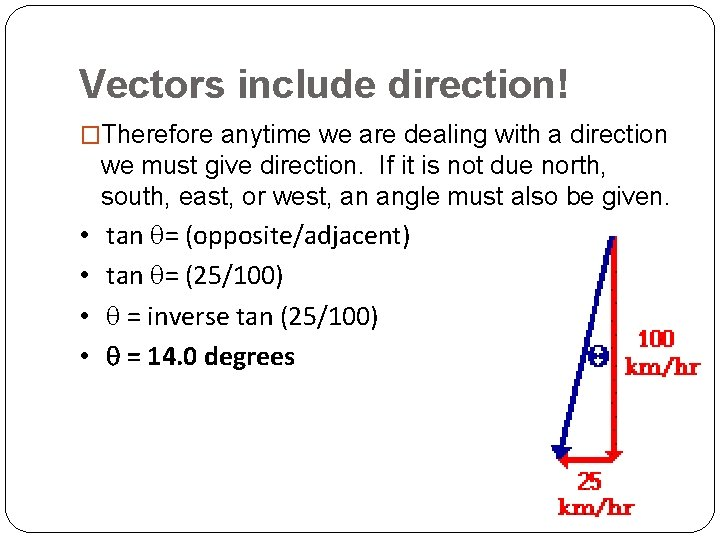Vectors include direction! �Therefore anytime we are dealing with a direction we must give direction. If it is not due north, south, east, or west, an angle must also be given. • • tan q= (opposite/adjacent) tan q= (25/100) q = inverse tan (25/100) q = 14. 0 degrees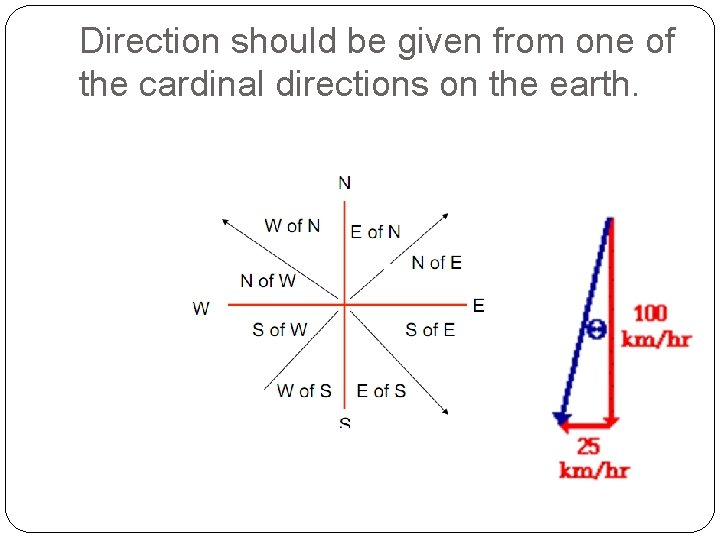Direction should be given from one of the cardinal directions on the earth.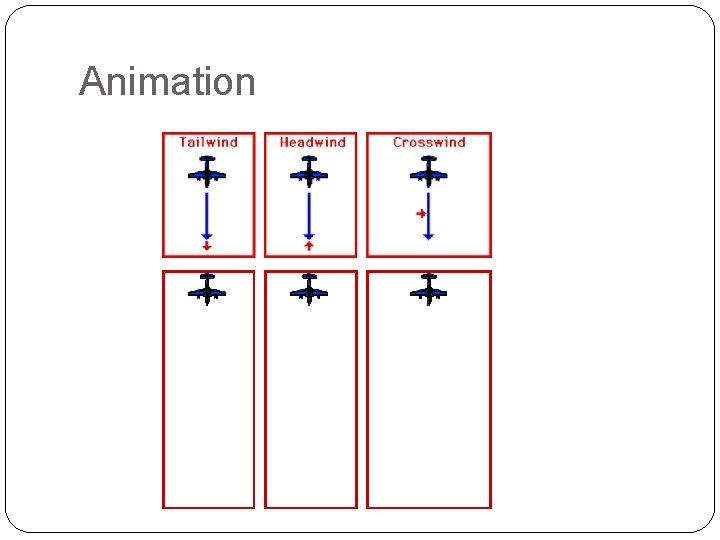Animation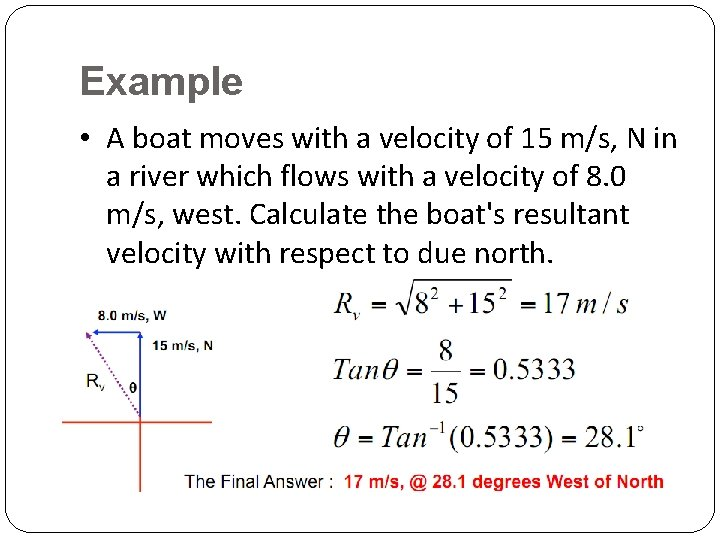Example • A boat moves with a velocity of 15 m/s, N in a river which flows with a velocity of 8. 0 m/s, west. Calculate the boat's resultant velocity with respect to due north.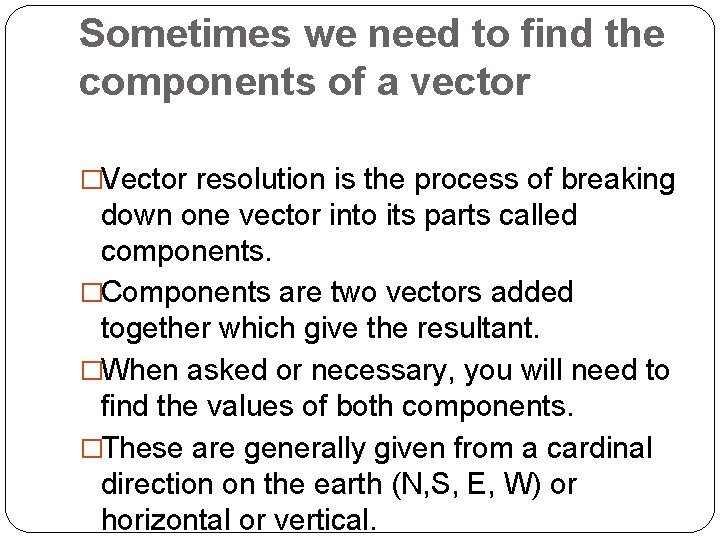Sometimes we need to find the components of a vector �Vector resolution is the process of breaking down one vector into its parts called components. �Components are two vectors added together which give the resultant. �When asked or necessary, you will need to find the values of both components. �These are generally given from a cardinal direction on the earth (N, S, E, W) or horizontal or vertical.Example • A plane moves with a velocity of 63. 5 m/s at 32 degrees South of East. Calculate the plane's horizontal and vertical velocity components.